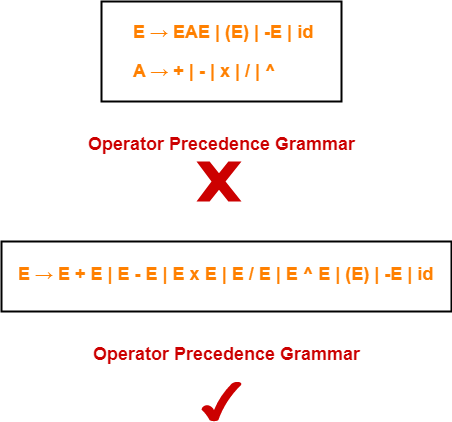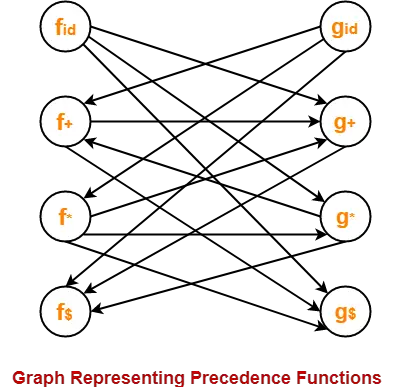# Operator Precedence Parsing

## Operator Precedence Grammar-

 A grammar that satisfies the following 2 conditions is called as Operator Precedence Grammar– There exists no production rule which contains ε on its RHS. There exists no production rule which contains two non-terminals adjacent to each other on its RHS.

• It represents a small class of grammar.
• But it is an important class because of its widespread applications.

### Examples-## Operator Precedence Parser-

 A parser that reads and understand an operator precedence grammar is called as Operator Precedence Parser.

## Designing Operator Precedence Parser-

In operator precedence parsing,

• Firstly, we define precedence relations between every pair of terminal symbols.
• Secondly, we construct an operator precedence table.

## Defining Precedence Relations-

The precedence relations are defined using the following rules-

### Rule-01:

• If precedence of b is higher than precedence of a, then we define a < b
• If precedence of b is same as precedence of a, then we define a = b
• If precedence of b is lower than precedence of a, then we define a > b

### Rule-02:

• An identifier is always given the higher precedence than any other symbol.
• \$ symbol is always given the lowest precedence.

### Rule-03:

• If two operators have the same precedence, then we go by checking their associativity.

## Parsing A Given String-

The given input string is parsed using the following steps-

### Step-01:

Insert the following-

• \$ symbol at the beginning and ending of the input string.
• Precedence operator between every two symbols of the string by referring the operator precedence table.

### Step-02:

• Start scanning the string from LHS in the forward direction until > symbol is encountered.
• Keep a pointer on that location.

### Step-03:

• Start scanning the string from RHS in the backward direction until < symbol is encountered.
• Keep a pointer on that location.

### Step-04:

• Everything that lies in the middle of < and > forms the handle.
• Replace the handle with the head of the respective production.

### Step-05:

Keep repeating the cycle from Step-02 to Step-04 until the start symbol is reached.

The advantages of operator precedence parsing are-

• The implementation is very easy and simple.
• The parser is quite powerful for expressions in programming languages.

The disadvantages of operator precedence parsing are-

• The handling of tokens known to have two different precedence becomes difficult.
• Only small class of grammars can be parsed using this parser.

## Important Note-

• In practice, operator precedence table is not stored by the operator precedence parsers.
• This is because it occupies the large space.
• Instead, operator precedence parsers are implemented in a very unique style.
• They are implemented using operator precedence functions.

## Operator Precedence Functions-

 Precedence functions perform the mapping of terminal symbols to the integers.

• To decide the precedence relation between symbols, a numerical comparison is performed.
• It reduces the space complexity to a large extent.

## Problem-01:

Consider the following grammar-

E → EAE | id

A → + | x

Construct the operator precedence parser and parse the string id + id x id.

## Solution-

### Step-01:

We convert the given grammar into operator precedence grammar.

The equivalent operator precedence grammar is-

E → E + E | E x E | id

### Step-02:

The terminal symbols in the grammar are { id, + , x , \$ }

We construct the operator precedence table as-

 id + x \$ id > > > + < > < > x < > > > \$ < < <

### Parsing Given String-

Given string to be parsed is id + id x id.

We follow the following steps to parse the given string-

### Step-01:

We insert \$ symbol at both ends of the string as-

\$ id + id x id \$

We insert precedence operators between the string symbols as-

\$ < id > + < id > x < id > \$

### Step-02:

We scan and parse the string as-

\$ < id > + < id > x < id > \$

\$ E + < id > x < id > \$

\$ E + E x < id > \$

\$ E + E x E \$

\$ + x \$

\$ < + < x > \$

\$ < + > \$

\$ \$

## Problem-02:

Consider the following grammar-

S → ( L ) | a

L → L , S | S

Construct the operator precedence parser and parse the string ( a , ( a , a ) ).

## Solution-

The terminal symbols in the grammar are { ( , ) , a , , }

We construct the operator precedence table as-

 a ( ) , \$ a > > > > ( < > > > > ) < > > > > , < < > > > \$ < < < <

### Parsing Given String-

Given string to be parsed is ( a , ( a , a ) ).

We follow the following steps to parse the given string-

### Step-01:

We insert \$ symbol at both ends of the string as-

\$ ( a , ( a , a ) ) \$

We insert precedence operators between the string symbols as-

\$ < ( < a > , < ( < a > , < a > ) > ) > \$

### Step-02:

We scan and parse the string as-

\$ < ( < a > , < ( < a > , < a > ) > ) > \$

\$ < ( S , < ( < a > , < a > ) > ) > \$

\$ < ( S , < ( S , < a > ) > ) > \$

\$ < ( S , < ( S , S ) > ) > \$

\$ < ( S , < ( L , S ) > ) > \$

\$ < ( S , < ( L ) > ) > \$

\$ < ( S , S ) > \$

\$ < ( L , S ) > \$

\$ < ( L ) > \$

\$ < S > \$

\$ \$

## Problem-03:

Consider the following grammar-

E → E + E | E x E | id

1. Construct Operator Precedence Parser.
2. Find the Operator Precedence Functions.

## Solution-

The terminal symbols in the grammar are { + , x , id , \$ }

We construct the operator precedence table as-

 g → f ↓ id + x \$ id > > > + < > < > x < > > > \$ < < <

#### Operator Precedence Table

The graph representing the precedence functions is-Here, the longest paths are-

• fid → gx → f+ → g+ → f\$
• gid → fx → gx → f+ → g+ → f\$

The resulting precedence functions are-

 + x id \$ f 2 4 4 0 g 1 3 5 0

To gain better understanding about Operator Precedence Parsing,

Watch this Video LectureGet more notes and other study material of Compiler Design.

Watch video lectures by visiting our YouTube channel LearnVidFun.

SummaryArticle Name
Operator Precedence Parsing
Description
In Compiler design, Operator Precedence Parser is a bottom-up parser that reads and understand Operator Precedence Grammar. Operator Precedence Parsing is simple and easy to use.
Author
Publisher Name
Gate Vidyalay
Publisher Logo